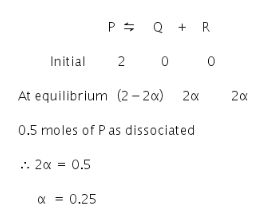# For the reaction: P==Q+R.Initially, 2 moles of P was taken

For the reaction: P==Q+R.Initially, 2 moles of P was taken. Up to equilibrium 0.5 moles of P was dissociated. What would be the degree of dissociation

Given:
Initial moles of P = 2 mole
Moles dissociated up to equilibrium = 0.5 moles
The equation is;The degree of dissociation is 0.25